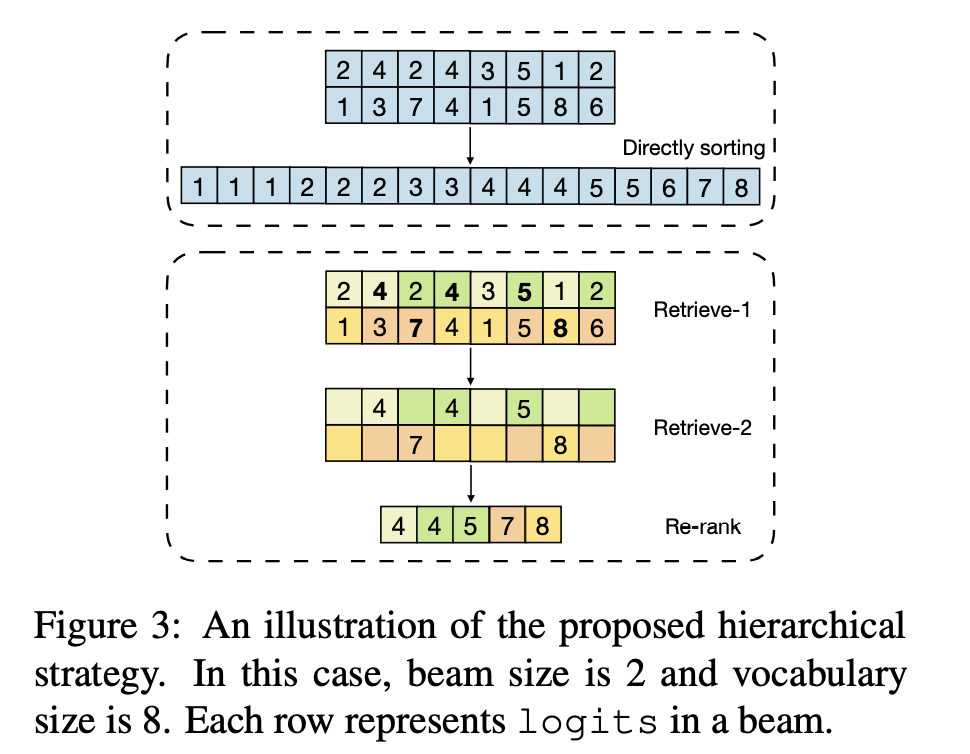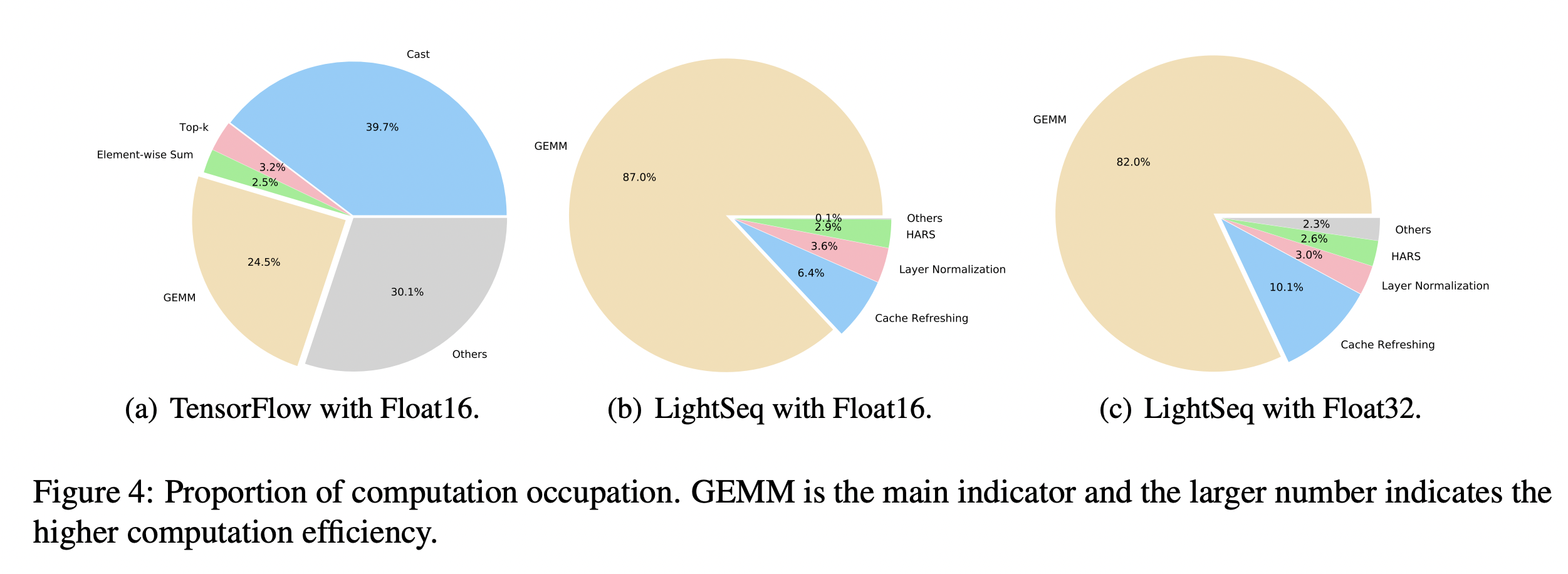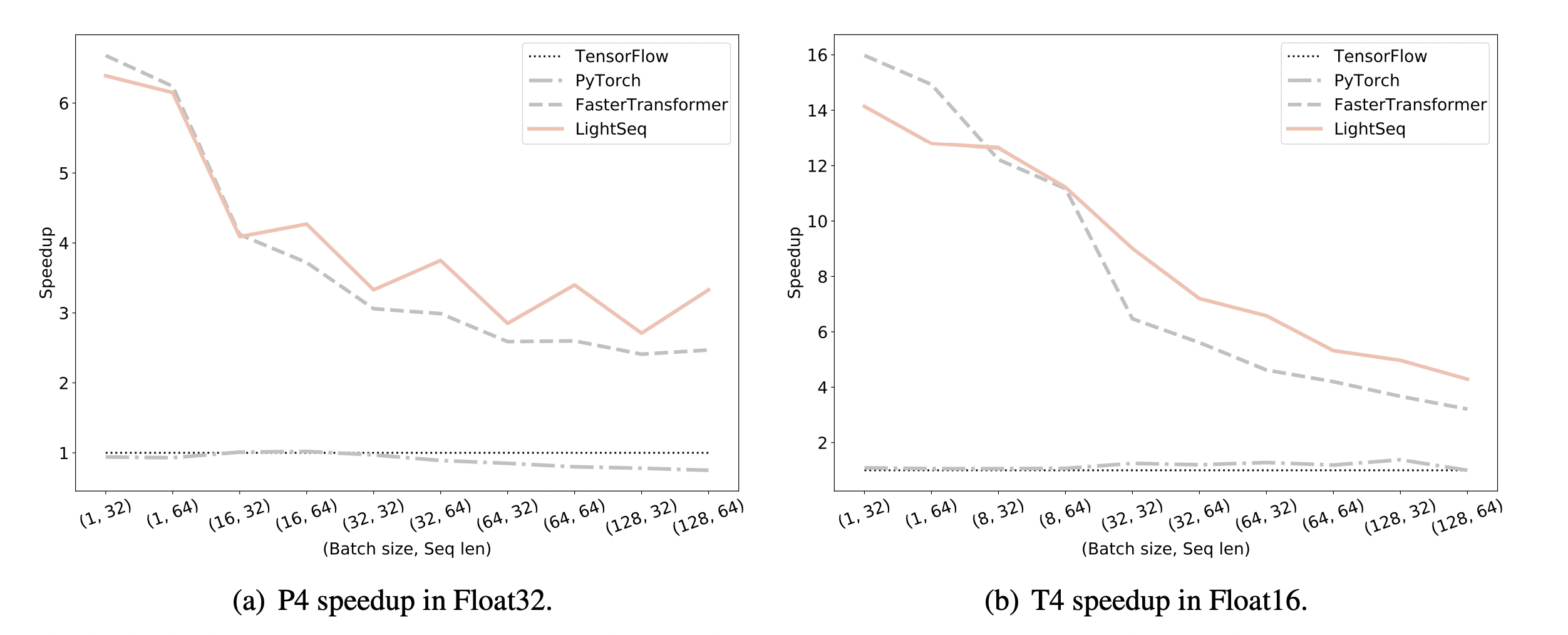# LightSeq Transformer高性能加速库

### 引言¶

Transformer，Bert模型在NLP领域取得巨大成功，得到广泛应用。而Transformer系列模型大小通常很大，在应用层提供相应服务是一个巨大的挑战。

### 介绍¶

1. 我们将Tensorflow/Pytorch实现中的一些细粒度Kernel，进一步融合实现成一个粗粒度的Kernel，从而避免大量核函数启动和GPU memory IO带来的时间成本

2. 我们设计了一种hierarchical（层级） auto regressive search来替代auto regressive search，进一步加速

3. 我们提出了一种动态显存复用策略，在NLP处理中，我们经常会遇到变长数据，给内存分配带来了困难。LightSeq预先定义了每个kernel最大可使用显存，并给不存在依赖关系的kernel进行共享，能够减少8倍内存分配。

### 方法¶Transformer主要分为两部分，特征计算层和输出层。特征计算层就是自注意力机制+FFN这些，而输出层则会随着任务不同而有些许改变。在NLU上是分类，而在NLG上是搜索(用beam search)。

### kernel fusion¶

template <typename T>
__global__ void ker_layer_norm(T *ln_res, T *vars, T *means, const T *inp,
const T *scale, const T *bias, int hidden_size) {
// step 0. compute local sum
float l_sum = 0;
float l_square_sum = 0;
const float4 *inp_f4 = (const float4 *)inp + blockIdx.x * hidden_size;
for (uint idx = threadIdx.x; idx < hidden_size; idx += blockDim.x) {
float4 val = inp_f4[idx];
l_sum += val.x + val.y + val.z + val.w;
l_square_sum +=
val.x * val.x + val.y * val.y + val.z * val.z + val.w * val.w;
}


// step 1. compute reduce sum
float mean_dim = float(hidden_size) * 4.f;
float reduce_val = {l_sum, l_square_sum};
blockReduce<ReduceType::kSum, 2>(reduce_val);
__shared__ float s_mean, s_var;
if (threadIdx.x == 0) {
s_mean = reduce_val / mean_dim;
if (means != nullptr) {
means[blockIdx.x] = s_mean;
}
s_var = reduce_val / mean_dim - s_mean * s_mean + LN_EPSILON;
vars[blockIdx.x] = s_var;
s_var = rsqrtf(s_var);
}


D(x) = E(X^2) - E(X)^2

// step 2. layer norm result
float4 *output_f4 = (float4 *)ln_res + blockIdx.x * hidden_size;
for (uint idx = threadIdx.x; idx < hidden_size; idx += blockDim.x) {
float4 vscale = __ldg((const float4 *)scale + idx);
float4 vbias = __ldg((const float4 *)bias + idx);
float4 val = inp_f4[idx];
val.x = (val.x - s_mean) * s_var * vscale.x + vbias.x;
val.y = (val.y - s_mean) * s_var * vscale.y + vbias.y;
val.z = (val.z - s_mean) * s_var * vscale.z + vbias.z;
val.w = (val.w - s_mean) * s_var * vscale.w + vbias.w;
output_f4[idx] = val;
}


auto regressive search方法如beam seach, diverse beam search等方法计算过于复杂。通常我们只需要置信度较高的几个label/tokens，而不是所有token都需要参与最终输出的计算。### 动态显存复用¶

LightSeq预先定义好动态shape的最大长度，在一开始先分配好最大显存，此外GPU显存将共享给不存在依赖关系的中间结果。

### 结果¶### 引言¶

Transformer，Bert模型在NLP领域取得巨大成功，得到广泛应用。而Transformer系列模型大小通常很大，在应用层提供相应服务是一个巨大的挑战。

### 介绍¶

1. 我们将Tensorflow/Pytorch实现中的一些细粒度Kernel，进一步融合实现成一个粗粒度的Kernel，从而避免大量核函数启动和GPU memory IO带来的时间成本
2. 我们设计了一种hierarchical（层级） auto regressive search来替代auto regressive search，进一步加速
3. 我们提出了一种动态显存复用策略，在NLP处理中，我们经常会遇到变长数据，给内存分配带来了困难。LightSeq预先定义了每个kernel最大可使用显存，并给不存在依赖关系的kernel进行共享，能够减少8倍内存分配。

### 方法¶Transformer主要分为两部分，特征计算层和输出层。特征计算层就是自注意力机制+FFN这些，而输出层则会随着任务不同而有些许改变。在NLU上是分类，而在NLG上是搜索(用beam search)。

### kernel fusion¶

template <typename T>
__global__ void ker_layer_norm(T *ln_res, T *vars, T *means, const T *inp,
const T *scale, const T *bias, int hidden_size) {
// step 0. compute local sum
float l_sum = 0;
float l_square_sum = 0;
const float4 *inp_f4 = (const float4 *)inp + blockIdx.x * hidden_size;
for (uint idx = threadIdx.x; idx < hidden_size; idx += blockDim.x) {
float4 val = inp_f4[idx];
l_sum += val.x + val.y + val.z + val.w;
l_square_sum +=
val.x * val.x + val.y * val.y + val.z * val.z + val.w * val.w;
}


// step 1. compute reduce sum
float mean_dim = float(hidden_size) * 4.f;
float reduce_val = {l_sum, l_square_sum};
blockReduce<ReduceType::kSum, 2>(reduce_val);
__shared__ float s_mean, s_var;
if (threadIdx.x == 0) {
s_mean = reduce_val / mean_dim;
if (means != nullptr) {
means[blockIdx.x] = s_mean;
}
s_var = reduce_val / mean_dim - s_mean * s_mean + LN_EPSILON;
vars[blockIdx.x] = s_var;
s_var = rsqrtf(s_var);
}


D(x) = E(X^2) - E(X)^2

// step 2. layer norm result
float4 *output_f4 = (float4 *)ln_res + blockIdx.x * hidden_size;
for (uint idx = threadIdx.x; idx < hidden_size; idx += blockDim.x) {
float4 vscale = __ldg((const float4 *)scale + idx);
float4 vbias = __ldg((const float4 *)bias + idx);
float4 val = inp_f4[idx];
val.x = (val.x - s_mean) * s_var * vscale.x + vbias.x;
val.y = (val.y - s_mean) * s_var * vscale.y + vbias.y;
val.z = (val.z - s_mean) * s_var * vscale.z + vbias.z;
val.w = (val.w - s_mean) * s_var * vscale.w + vbias.w;
output_f4[idx] = val;
}


### Hierarchical Auto Regressive Search¶

auto regressive search方法如beam seach, diverse beam search等方法计算过于复杂。通常我们只需要置信度较高的几个label/tokens，而不是所有token都需要参与最终输出的计算。

1. 使用softmax计算，并将结果写入gpu memory
2. 读取结果，并从中选取top-K的beams和tokens

1. 随机将logits组分到k个组
2. 计算每个group($g_i$)的最大值，记为$m_i$
3. $m_i$中，计算最小值$R$，可以看作是logits粗略的top-k值
4. 选择值大于R的logits，并写入到gpu memory### 动态显存复用¶

LightSeq预先定义好动态shape的最大长度，在一开始先分配好最大显存，此外GPU显存将共享给不存在依赖关系的中间结果。

### 结果¶